# MCAT Physical : Oxidation Number

## Example Questions

← Previous 1

### Example Question #1 : Oxidation Number

What is the oxidation state of sulfur in H2SO4?

+4

0

-5

+6

-2

+6

Explanation:

Since there is no overall charge on the compound the oxidation states must cancel out. Although the oxidation state of hydrides are +1, there are two in the compound that must be accounted for. The same is true for oxygen; although the oxidation number of oxygen is -2, there are four oxygens present accounting for a total of -8. The +2 state contribution from the hydrides and -8 from the oxygens results in a -6 charge. The oxidation state on sulfur must be +6 for the molecule to be neutral.

### Example Question #1 : Oxidation Number

What is the oxidation number of chromium in calcium dichromate (CaCr2O7)?

+7

+2

+6

-2

0

+6

Explanation:

Since the calcium ion has a charge of +2, the dichromate anion must have a charge of -2. Oxygen always has an oxidation number of -2, so the seven oxygens in the molecule have a total number of -14. That leaves a charge of +12 that the two chromiums must balance out (-2 - (-14) = 12). Each chromium must therefore have an oxidation number of +6.

### Example Question #3 : Oxidation Number

What is the oxidation state of nitrogen in HNO3?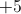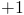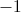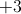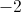Explanation:

When applying oxidation numbers there are certain hierarchical rules that must be followed.

1. The sum of oxidation states of all the elements in a molecule must add up to the overall charge.

2. Group 1 and Group 2 elements have +1 and +2 oxidation states, respectively.

3. Fluorine has an oxidation state of –1.

4. Hydrogen has an oxidation state of +1 (except in metal hydrides).

5. Oxygen has an oxidation state of –2.

6. Elements of the same group (excluding transition metals) generally have the same oxidation state.

When applying the oxidation state to HNO3, hydrogen has a +1 oxidation state, and each of the oxygen molecules has a –2 oxidation state. Since there is only one hydrogen molecule and three oxygen molecules, the oxidation state of nitrogen must balance out the charge of the hydrogen molecule and oxygen molecules combined.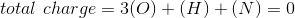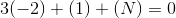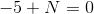Nitrogen must have a +5 oxidation state.

### Example Question #1 : Oxidation Number

What is the oxidation number of hydrogen in NaH?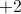Explanation:

When applying oxidation numbers there are certain hierarchical rules that must be followed.

1. The sum of oxidation states of all the elements in a molecule must add up to the overall charge.

2. Group 1 and Group 2 elements have +1 and +2 oxidation states, respectively.

3. Fluorine has an oxidation state of –1.

4. Hydrogen has an oxidation state of +1 (except in metal hydrides).

5. Oxygen has an oxidation state of –2.

6. Elements of the same group (excluding transition metals) generally have the same oxidation state.

Following the oxidation rules, group 1 elements must have an oxidation number of +1.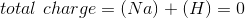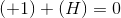To balance out the overall charge of zero, the hydrogen in this compound must have an oxidation state of –1. Note that NaH is a metal hydride; therefore, the oxidation state of hydrogen is not necessarily +1.

### Example Question #1 : Oxidation Number

What is the correct oxidation number of zinc in the compound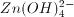?

0

-1

+1

-2

+2

+2

Explanation:

The sum of oxidation numbers of each element in this compound must add to the total charge of -2. Hydrogen always has an oxidation number of +1, and oxygen always has an oxidation number of -2.

So, in total for oxygen we have (4) * (-2) = -8, and for hydrogen we have (4) * (+1) = +4.

Combining the oxygen and hydrogen, (+4) + (-8) = -4, so we need an additional +2 to achieve the total charge of -2. Zn must provide this balancing charge, and have an oxidation number of +2.

### Example Question #6 : Oxidation Number

Which of the following statements between HClO4 and HClO3 is true?

The oxidation number of chlorine in HClO4 has been decreased in HClO3

The oxidation number for chlorine in HClO4 has increased in HClO3

The oxidation numbers for all atoms are the same in both molecules

The oxidation number of oxygen in HClO4 has been decreased in HClO3

The oxidation number of chlorine in HClO4 has been decreased in HClO3

Explanation:

Oxidation number is a concept that you will also encounter in electrochemistry lectures. It is used to track electrons in an oxidation-reduction reaction. Overall, the sum of the oxidation states of all of the atoms in the molecule must equal the overall charge on the molecule. It is important to remember some of the commonly accepted oxidation states for atoms, so that you can predict the oxidation numbers on other atoms. Here are some key oxidation numbers useful to know for the MCAT:

1. Atoms in elemental form have an oxidation state of 0.

2. Halogens are commonly given an oxidation state of –1.

3. Hydrogen and alkali metals are commonly given an oxidation state of +1.

4. Oxygen is commonly given an oxidation number of –2.

Keeping these rules in mind, we can assume that the hydrogens and oxygens in the two compounds maintain the same oxidation state. Chlorine, however, is attached to one less oxygen in HClO3. As a result, the oxidation state of Cl has decreased from +7 to +5, when comparing HClO4 to HClO3.

### Example Question #1 : Oxidation Number

Which element in the given compounds has the highest oxidation number?

Sulfur in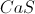Sulfur in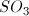Carbon in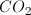Oxygen in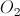Aluminum in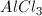Sulfur inExplanation:

We can calculate the oxidation number in each answer to find the greatest value.

In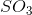, oxygen will have a value ofand the molecule is neutral. Sulfur must have an oxidation number of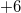to balance the three oxygens.

In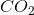, oxygen will have a value ofand the molecule is neutral. Carbon must have an oxidation number of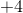to balance the two oxygens.

In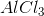, chlorine will have a value ofand the molecule is neutral. Aluminum must have an oxidation number of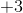to balance the three chlorines.

In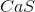, calcium will have a value ofand the molecule is neutral. Sulfur must have an oxidation number of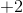to balance the calcium.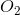is a molecule comprised of a single element; each atom will have an oxidation number of.

### Example Question #8 : Oxidation Number

What is the oxidation number of chlorine in the perchlorate ion?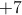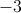Explanation:

To find the oxidation number, first remember that the total charge must be preserved. The total charge of the perchlorate ion is.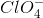Each oxygen will have an oxidation number of. The oxidation of chlorine must be used to balance this negative charge.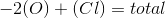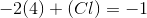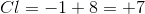### Example Question #9 : Oxidation Number

Which molecule contains an atom with an oxidation state of?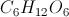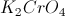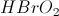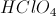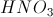Explanation:

The rules for oxidation state note that certain atoms will have constant oxidation values, while others are more likely to vary. Oxygen, for example, will always have an oxidation state of, alkali metals will always have an oxidation state of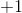, and hydrogen will (almost) always have an oxidation state of.

All of the given compounds are neutral, meaning that their oxidation numbers must sum up to zero. Using our listed rules to look at these molecules, we can try to find any missing values.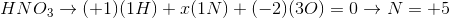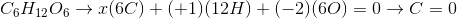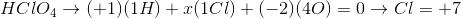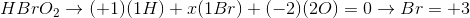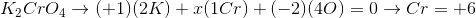Chromium in potassium dichromate is the only atom in this set to have an oxidation state of.

### Example Question #10 : Oxidation Number

What is the difference in oxidation number between manganese in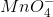and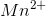?Explanation:

The sum of the oxidation numbers of each atom in a molecule must equal the net charge of the molecule.

Oxygen will always have an oxidation number of. Inwe can find the oxidation number of manganese with the following equation: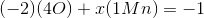Solving this out, we can see that the charge on manganese must be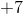.

In the manganese ions, there is only one atom and the net charge is. The oxidation of this manganese atom must be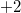to balance the ionic charge.

The difference between these oxidation numbers is: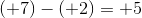← Previous 1